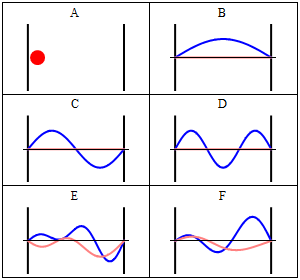# Infinite Square WellA particle of mass $m$ is in an infinite square well of width $a$. The particle is equally likely to be found in the region. What is the probability that a measurement of energy is found at ground state?

×